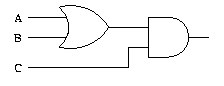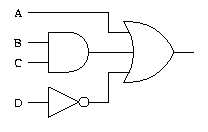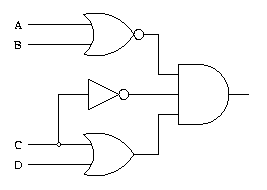Logic Gate ExamplesHere are some logic gate circuit problems:
• Draw a logic circuit for (A + B)C.• Draw a logic circuit for A + BC + D.• Draw a logic circuit for AB + AC.• Draw a logic circuit for (A + B)(C + D)C.